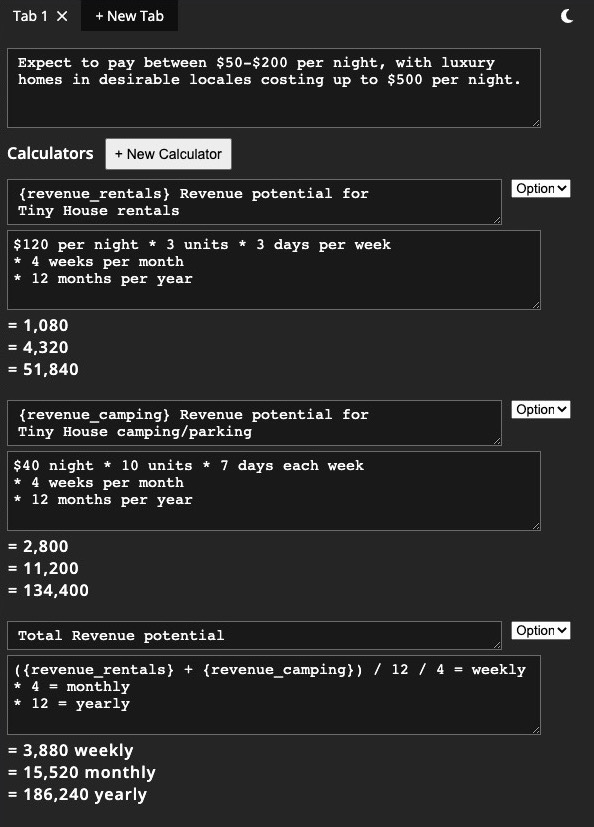Tab 1
+ New Tab
Calculators

## Instructions

(See screenshot below for full example)

### Overview

Freely type math expressions, line by line, and optionally add text to indicate what numbers mean.
Example:
$257 flights +$54 ride to airport
/ 2 ppl

### Calculation Names & Variables

Above each calculator is a Calculation Name box, where you can enter a name for the calculation. Calculation Names can be referenced in other calculators by wrapping the name in {brackets}.
Example:
Calculator Name box: construction_cost

### Calculation Names - Multiple Lines

To increase the height of a Calculation Name box, enter a new line.

### Result Output Text

To have text appear with the current line in the result output, add = before it.
Example:
$500 -$35.60
/ 3 = per person
(below screenshot shows how this looks)

### Example Screenshot